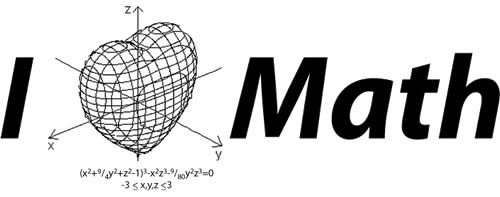## A Belated Valentine’s Day Post

This is romantic!  So listen up!

A 3D heart shape may be drawn using the following implicit function:Or, in Python:

```def  heart_3d(x,y,z):
return (x**2+(9/4)*y**2+z**2-1)**3-x**2*z**3-(9/80)*y**2*z**3```

Trouble is, there is no direct way of graphing implicit functions in Python. But anything can be found on Stack Overflow.

Putting it all together:

```#!/usr/bin/env python
from mpl_toolkits.mplot3d import Axes3D
from matplotlib import cm
from matplotlib.ticker import LinearLocator, FormatStrFormatter
import matplotlib.pyplot as plt
import numpy as np
def heart_3d(x,y,z):
return (x**2+(9/4)*y**2+z**2-1)**3-x**2*z**3-(9/80)*y**2*z**3

def plot_implicit(fn, bbox=(-1.5,1.5)):
''' create a plot of an implicit function
fn  ...implicit function (plot where fn==0)
bbox ..the x,y,and z limits of plotted interval'''
xmin, xmax, ymin, ymax, zmin, zmax = bbox*3
fig = plt.figure()
A = np.linspace(xmin, xmax, 100) # resolution of the contour
B = np.linspace(xmin, xmax, 40) # number of slices
A1,A2 = np.meshgrid(A,A) # grid on which the contour is plotted

for z in B: # plot contours in the XY plane
X,Y = A1,A2
Z = fn(X,Y,z)
cset = ax.contour(X, Y, Z+z, [z], zdir='z',colors=('r',))
# [z] defines the only level to plot for this contour for this value of z

for y in B: # plot contours in the XZ plane
X,Z = A1,A2
Y = fn(X,y,Z)
cset = ax.contour(X, Y+y, Z, [y], zdir='y',colors=('red',))

for x in B: # plot contours in the YZ plane
Y,Z = A1,A2
X = fn(x,Y,Z)
cset = ax.contour(X+x, Y, Z, [x], zdir='x',colors=('red',))

# must set plot limits because the contour will likely extend
# way beyond the displayed level.  Otherwise matplotlib extends the plot limits
# to encompass all values in the contour.
ax.set_zlim3d(zmin,zmax)
ax.set_xlim3d(xmin,xmax)
ax.set_ylim3d(ymin,ymax)

plt.show()

if __name__ == '__main__':
plot_implicit(heart_3d)```

Show this to your date on the next Valentine’s Day, because it is too late for this one. Trust me, results are guranteed. Not sure what kind of results though.

### 2 Responses to “A Belated Valentine’s Day Post”

1.Julien says:

2.Iddo says: